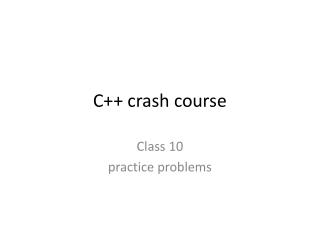Download PresentationC++ crash course

# C++ crash course

Download Presentation## C++ crash course

- - - - - - - - - - - - - - - - - - - - - - - - - - - E N D - - - - - - - - - - - - - - - - - - - - - - - - - - -
##### Presentation Transcript

1. C++ crash course Class 10 practice problems

2. Pointers The following function is trying to swap the contents of two variables. Why isn’t it working? void swap(int &a, int b) { inttmp = a; a = b; b = tmp; }

3. Strings • Write a function which, given a char array and two ints, will return a string * which holds the section between the two ints. Return the null pointer if it’s not possible. string *substr(char arr[], int start, int stop);

4. Dynamic Memory Allocation • The below is bad practice. Why is that? int *p = new int(5); int j = 7; p = &j;

5. Pointers • This won’t compile. Why? double a = 10; int *aptr = &a;

6. Pointers • What is “this”, and what is its use?

7. Data Types • How is initializing a vector of ints different from initializing a single int?

8. Data Types • Write the following in decimal. 1010012 0456 0x21

9. Files and Unix • What’s the difference between > and < on the Unix command line?

10. What’s the output? int size = 3; intarr = {7, 2, 4}; char name = “Jeff Jones”; int *iptr; char *cptr; iptr = arr; cout << iptr << “ and “ << *iptr << endl; cptr = &name; cout << cptr << endl; cout << *(cptr + 3) << “ and “ << *(cptr-4) << endl; cptr ++; cout << name << “ and “ << cptr << endl;

11. Operators • What do all of the following do? *itr++; *++itr; (*itr)++;

12. What does this output? void funct(int a); int main() { int a = 5, *b = &a, c[] = {10, 21, 12}; int *d, *e, f; cout << *b << “ “ << *c + 1 << endl; e = b; b = new int; d = new int; *d = 7; *b = 9; *e = 11; cout << *e << *d << a; funct(a); e = b; free(b); cout << *e << f << a; return 0; }

13. Scope • What’s the scope operator (::) doing in these instances? std::cout intAnimal_type::avg_age() const What can you do to avoid using the scope operator repeatedly?

14. Overloading Operators • What’s the function prototype to overload the output operator? • return type • function name • parameter list

15. const • What is the purpose of the “const” keyword? • const gets a little tricky with pointers. Give an example.

16. Loops • Write a function to sum even numbers from int a to int b, and return the result.

17. Reserved Words • What are reserved words? Give 3 examples.

18. Functions • What’s the difference between int a, int &a, and int *a in a function parameter list?

19. ? • What are these called? What do they do? #include #define #ifndef #endif

20. Operators • What is precedence? What is associativity? • Is assignment left or right associative? • Is arithmetic left or right associative?

21. Sorting • Sort these using insertion sort, and show all the steps. 5 4 15 99 46 82 93 54 71 26 12

22. Sorting • Write an insertion sort on a vector of int *.

23. Classes • What’s a constructor? How do you identify one? • Write a default constructor for this class: class Mystery { int a; bool b; string s; };

24. Classes • What is the difference between public, private and friend?

25. Data Types • What’s the difference between a literal and a constant?